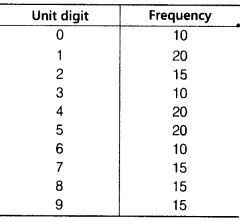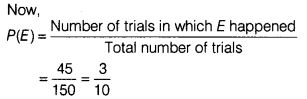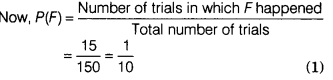# On one page of a telephone directory, there are 150 phone numbers

On one page of a telephone directory, there are 150 phone numbers. The frequency distribution of their units digit is given belowOne of the numbers is chosen at random from the page. What is the probability that the units digit of the chosen number is
(i) less than 3?
(ii) greater than 8?

Given, total number of phone numbers = 150
Total number of trials=150
(i) Let E be the event of getting the number with unit digit less than 3.
∴ Number of trials in which the event
E happened = 10 + 20 +15 = 45
Now,(ii) Let F be the event of getting number with unit digit greater than 8.
∴ Number of trials in which F happened = 15